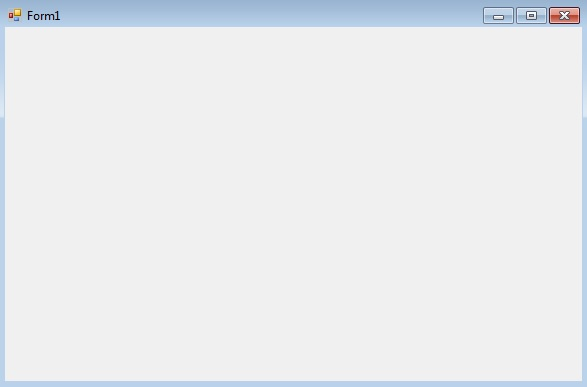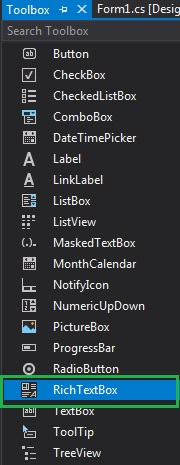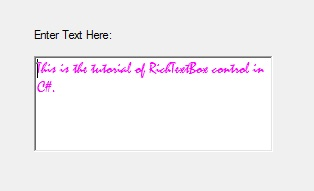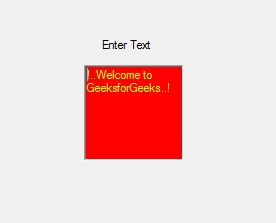# How to set the Foreground Color of the RichTextBox in C#?

In C#, RichTextBox control is a textbox which gives you rich text editing controls and advanced formatting features also includes a loading rich text format (RTF) files. Or in other words, RichTextBox controls allows you to display or edit flow content, including paragraphs, images, tables, etc. In RichTextBox, you are allowed to change the foreground color of the RichTextBox control using ForeColor Property which makes your RichTextBox control more attractive. You can set this property in two different ways:

1. Design-Time: It is the easiest way to set the foreground color of the RichTextBox as shown in the following steps:

• Step 1: Create a windows form as shown in the below image:
Visual Studio -> File -> New -> Project -> WindowsFormApp• Step 2: Drag the RichTextBox control from the ToolBox and drop it on the windows form. You are allowed to place a RichTextBox control anywhere on the windows form according to your need.• Step 3: After drag and drop you will go to the properties of the RichTextBox control set the foreground color of the RichTextBox control.2. Run-Time: It is a little bit trickier than the above method. In this method, you can set the foreground color of the RichTextBox control programmatically with the help of given syntax:

`public override System.Drawing.Color ForeColor { get; set; }`

Here, Color indicates the foreground color of the RichTextBox. The following steps show how to set the ForeColor property of the RichTextBox dynamically:

• Step 1: Create a RichTextBox using the RichTextBox() constructor is provided by the RichTextBox class.
```// Creating RichTextBox using RichTextBox class constructor
RichTextBox rbox = new RichTextBox();
```
• Step 2: After creating RichTextBox, set the ForeColor Property of the RichTextBox provided by the RichTextBox class.
```// Setting the foreground color
rbox.ForeColor = Color.Yellow;
```
• Step 3: And last add this RichTextBox control to the form using Add() method.
```// Add this RichTextBox to the form
```

Example:

 `using` `System; ` `using` `System.Collections.Generic; ` `using` `System.ComponentModel; ` `using` `System.Data; ` `using` `System.Drawing; ` `using` `System.Linq; ` `using` `System.Text; ` `using` `System.Threading.Tasks; ` `using` `System.Windows.Forms; ` ` `  `namespace` `WindowsFormsApp30 { ` ` `  `public` `partial` `class` `Form1 : Form { ` ` `  `    ``public` `Form1() ` `    ``{ ` `        ``InitializeComponent(); ` `    ``} ` ` `  `    ``private` `void` `Form1_Load(``object` `sender, EventArgs e) ` `    ``{ ` `        ``// Creating and setting the  ` `        ``// properties of the label ` `        ``Label lb = ``new` `Label(); ` `        ``lb.Location = ``new` `Point(251, 70); ` `        ``lb.Text = ``"Enter Text"``; ` ` `  `        ``// Adding this label in the form ` `        ``this``.Controls.Add(lb); ` ` `  `        ``// Creating and setting the  ` `        ``// properties of RichTextBox ` `        ``RichTextBox rbox = ``new` `RichTextBox(); ` `        ``rbox.Location = ``new` `Point(236, 97); ` `        ``rbox.BackColor = Color.Red; ` `        ``rbox.ForeColor = Color.Yellow; ` `        ``rbox.Text = ``"!..Welcome to GeeksforGeeks..!"``; ` ` `  `        ``// Adding this RichTextBox in the form ` `        ``this``.Controls.Add(rbox); ` `    ``} ` `} ` `} `

Output:Whether you're preparing for your first job interview or aiming to upskill in this ever-evolving tech landscape, GeeksforGeeks Courses are your key to success. We provide top-quality content at affordable prices, all geared towards accelerating your growth in a time-bound manner. Join the millions we've already empowered, and we're here to do the same for you. Don't miss out - check it out now!

Previous
Next